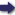## Bīrūnī, Muḥammad ibn Aḥmad, Alberuni's India (v. 2)

(London :  Kegan Paul, Trench, Trübner & Co.,  1910.)

 Tools

## Search this bookPrev Page 79 Next``` CHAPTER LV. 79 Lastly, there is the possibility that the minus belongs to the diameter of the moon. In that case the relation of ZX, which has been determined in yojanas, to SL, the yojanas of the true distance of the moon, is the same as the relation of ZX reckoned in minutes to SL, this being the sinus totus (conjectural rendering). By this method is found what Brahmagupta wants to find, quite correctly, without the division by the mean radius of the sphere of the moon, which is derived from the. yojanas of the sphere of heaven (v. p. 72). (For the last three passages vide Notes.) The methods of the computation of the diameters of Thecompu- -I • 1 j_n TT' J 1 tationofthe sun and moon, as given by the Hindu canones, such as diameters the Khandctkhddyaka and Karanctsdra, are the same as mooTac" are found in the canon of Alkhwarizmi. Also the com- to'^'tw to other sources. putation of the diameter of the shadow in the Khandct- khddyctkct is similar to that one given by Alkhwarizmi, whilst the Karctnasara has the following method:— " Multiply the bhukti of the moon by 4 and the bhukti of the sun by 13. Divide the difference between the two products by 30, and the quotient is the diameter of the shadow." The Kctrancttilctkct gives the following method for the Diameter of ■-. „, .._ the sun and computation of the diameter of the sun :—"Divide the ofthesha- ,,-,.„, , -, .-, ii-if>' *^°^ aocord- bhitkti of the sun by 2, and write down the half m two ing to the different places. In the one place divide it by 10, and laka' add the quotient to the number in the second place. The sum is the number of minutes of the diameter of the sun." In the computation of the diameter of the moon, he first takes the bhukti of the moon, adds thereto -gVth of it, and divides the number by 25. The quotient is the number of the minutes of the moon's diameter. In the computation of the diameter of the shadow, he multiplies the bhukti of the sun by 3, and from the product he subtracts •^V'^h of it. The remainder he sub¬ tracts from the bhukti of the moon, and the double of ```Prev Page 79 Next